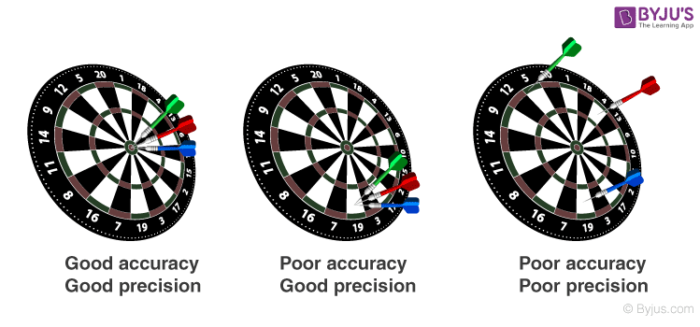# Difference Between Accuracy And Precision

When it comes to the matter of measuring an item or substance, the topic of discussion turns from the item to the accuracy or precision of the measurement. Both these terms are used interchangeably quite often, but they are not the same.

## What is Accuracy?

Accuracy would be referred to as the level of correctness of measurement when compared to its true value.

## What is Precision?

Precision, on the other hand, refers to the level of absolute exact the measurement is to the strictest manner.In simple terms, precision is the term used to see how reliable and consistent the measurement of the experiment is while accuracy on the other hand checks the closeness of the experiment measurement with the ideal or accepted value.

An ideal analogy to remember accuracy and precision is to remember a basketball player trying to shoot at the baskets where if the player would shoot with the most accuracy the ball would land either close or into the basket. If the player tried to shoot with precision, the ball would fall in the exact location which would be either close or not close to the basket. A right player would be accurate and precise at the same time by shooting the ball always into the basket.

## Difference Between Accuracy And Precision

 Basis Accuracy Precision Definition It could be defined as the level of correctness of a measurement to its true value. It could be defined as the sharp exactness of a measurement. Measurement Method Has one factor used for measuring. Has multiple factors for measurement. Mutual  Relationship Accurate items have to be precise in most cases. Precise items may or may not be accurate.

The points mentioned below are an indication of how terms like accuracy and precision vary from one another despite being used by people interchangeably on most occasions. For more information on topics like uncertainty in measurement, keep visiting BYJU’S.

Ans:  True.

### 2. How to determine accuracy?

Ans:  To measure accuracy follow the steps given below:
Step 1 – Get the difference value between accepted and the experimental value
Step 2 – Divide the difference value by the accepted value.

### 3. How to determine precision?

Ans:  To measure precision follow the below-given steps:
Step 1 – Sort the data and determine the highest and lowest measured value.
Step 2 -Take the difference between the lowest measured and highest measured value.

### 4. How to determine the percent error?

Ans:  To determine the percent error:
Step 1 – Take the difference between accepted value and experimental value.
Step 2 – Take the absolute value of step 1.
Step 3 – Divide the answer obtained from step 2 by the accepted value.
Step 4 – Multiply the answer got in step 3 by 100
Step 5 – Add percentage(%) symbol to indicate the answer in percentage

### 5. What is the difference between accuracy and precision ?

Ans:  Accuracy refers to the degree of closeness towards the true value and precision refers to the degree of repetition of the same value.

Test your Knowledge on Accuracy And Precision Difference

#### 1 Comment

1. Harshita

I like this educational platform, it’s very helpful to the struggling students .
I like this app!